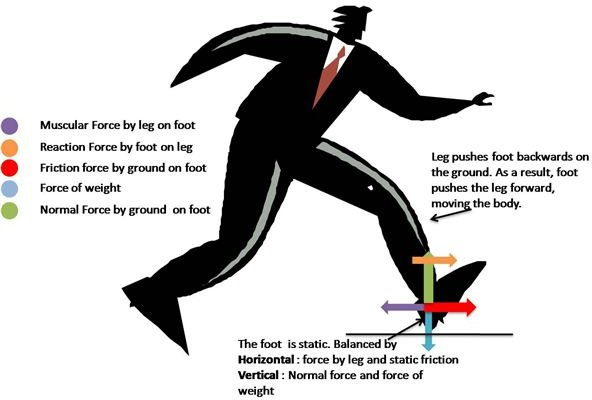# Application of Newton's Laws in Walking Including a Free Body Diagram

Page content

I have always been intrigued by the phenomenon of walking by humans. Discover the interplay of forces involved in walking to better understand the laws of motion and friction.

The following concepts explain the activity of walking:

1. Identifying the System
2. Free Body Diagram of the System
3. Newton’s third law of action and reaction
4. Static and dynamic friction

First and foremost, let us understand the chain of forces that lead to pushing the body forward is the push by the leg on the foot backwards.

• The work done in moving the body forward and dissipation as heat by friction is done by the backward pushing force of the leg muscles.
• The leg and the body moves forward but the foot remains stationary.
• The first figure below demonstrates the forces on the body as a whole. Here, the system is the entire body of the man, including the foot.
• The second figure shows all the forces on the foot and leg individually.

## Newton’s Laws of Motion Applied## Forces Involved

Let us consider the forces in detail.

• Equilibrium of the The Foot (System - The Foot): The leg pushes the foot backward. As the leg tries to move forward, the frictional force, in order to avoid the relative motion, acts in the forward direction on the foot. Assuming the ground is rough enough, the force manages to keep the foot in place. Since the foot does not move, the force is static friction and not dynamic friction. The foot is balanced horizontally by backwards muscular force of the leg and forward static friction from the ground.
• An unbalanced force on the leg and the rest of the body connected to it. (System - The Leg): As the leg pushes the foot forward, the foot, obeying the Newton’s law of action and reaction, pushes the leg forward. This is the unbalanced force on the foot that makes the body move.
• Considering the whole man (System - The Man): The muscular force of the leg muscles on the foot is an internal force for this system. The body, on the whole is unbalanced horizontally and the unbalanced force is the force of static friction.
• Therefore, the work in walking is done by the internal muscular forces of the leg.